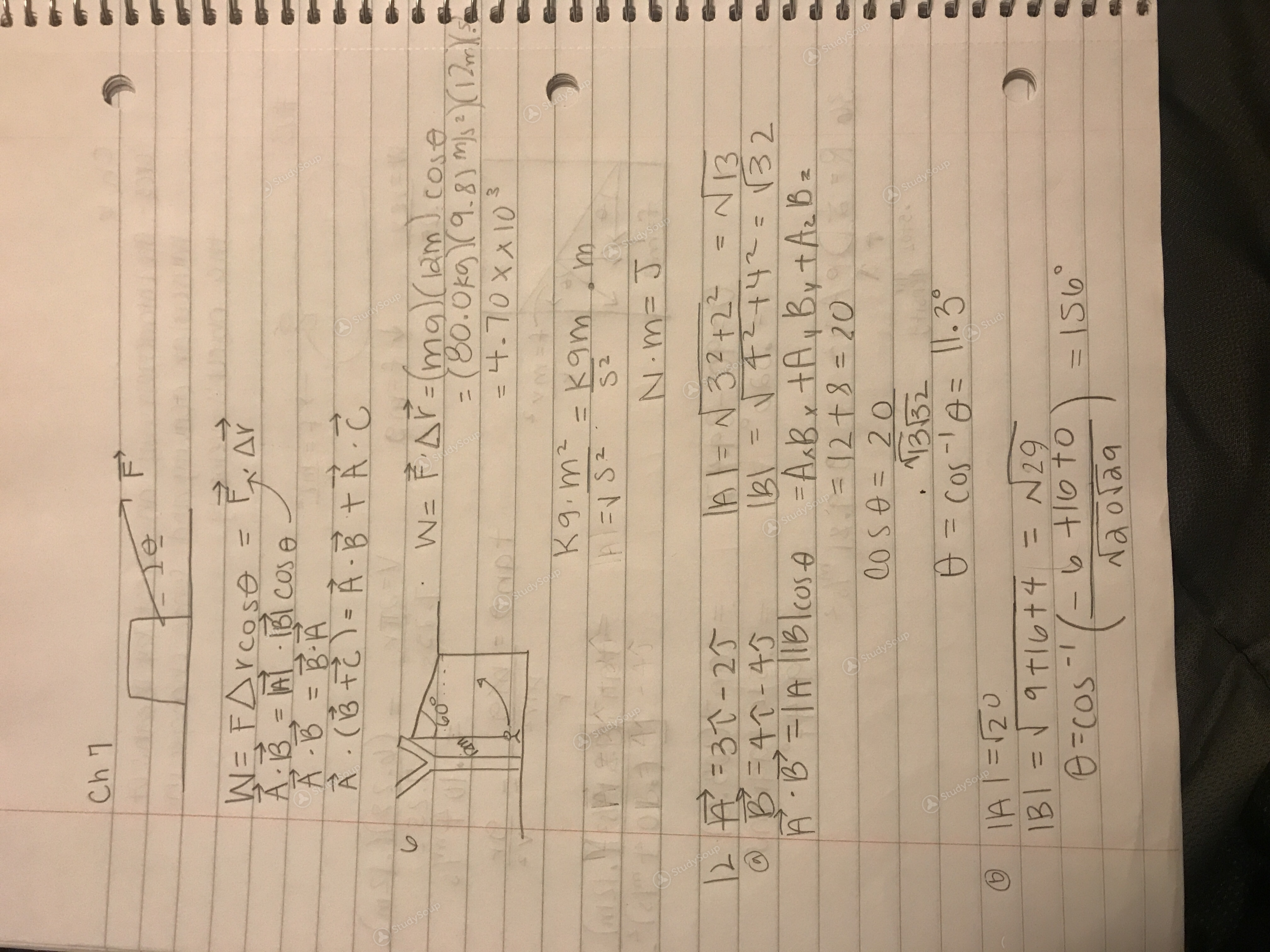Limited time offer 20% OFF StudySoup Subscription details

# UH - PHYS 1321 - Class Notes - Week 2

### Created by: Melii Elite Notetaker

> > > > UH - PHYS 1321 - Class Notes - Week 2

UH - PHYS 1321 - Class Notes - Week 2

This preview shows page 1 of a 2 page document. to view the rest of the contentThis is the end of the preview. Please to view the rest of the contentJoin more than 18,000+ college students at University of Houston who use StudySoup to get ahead
##### Description: Energy of a system
2 Pages 36 Views 28 Unlocks
• Notes, Study Guides, Flashcards + More!

Unformatted text preview: Chi U WE FA r cose = F Ar A,B,= A BICOS E S A - B = B-A A. (FE= -B F -E V W = FAF-mg Im J. Cose = (80.Oko (9.8) M 2 - 4.70xxl) 3 Kg.m2 = Kgm . m N.M= J 2 F. =31 - 25 B = N 32 +22= N 13 B = 40-45 alb\ = N 4294 = 32 A - B =[A IB\cos A = Ask, A, B, A. B = 12 +8 = 20 CO S A = 20 . 1332 6 = Cos-le= (1.39 O JA I = 120 IBI = 9 t|6+4 = N29 =cos -' ( b to na olag PRINSIPPET SPRIPPILIPPIJCR-4+4 = 3 B = 9 + S A = Cos'/ 0 - 13 - 82.3 3.5 W=FAT - dit f.dr W= Sem F'dx = STM Fax + = \2 N-m + |2N.m = 24Nm = a4J W = som far --3Nm --3J c) W = Fdx = .3 mEdx + Si =a4J + (-33) = 2 J As soup F AX =- srudysrup

Get Full Access to UH - PHYS 1321 - Class Notes - Week 2
×
Get Full Access to UH - PHYS 1321 - Class Notes - Week 2

I don't want to reset my password

Need help? Contact support

Need an Account? Is not associated with an account
We're here to help